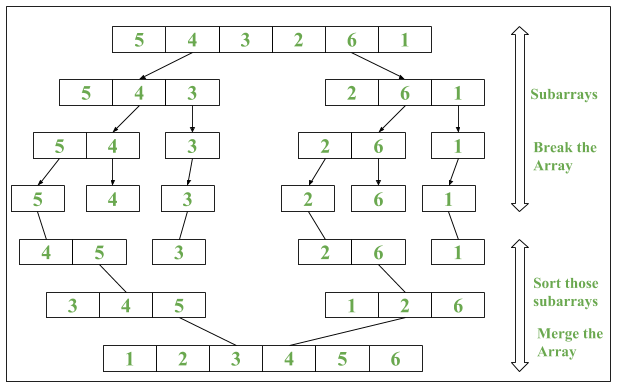# Java 8 | Arrays parallelSort() method with Examples

• Difficulty Level : Easy
• Last Updated : 11 Dec, 2018

Java 8 introduced a new method called as parallelSort() in java.util.Arrays Class. It uses Parallel Sorting of array elements

Algorithm of parallelSort()

```1. The array is divided into sub-arrays and that
sub-arrays is again divided into their sub-arrays,
until the minimum level of detail in a set of array.
2. Arrays are sorted individually by multiple thread.
3. The parallel sort uses Fork/Join Concept for sorting.
4. Sorted sub-arrays are then merged.```

Syntax :

1. For sorting data in ascending order :
```public static void parallelSort(Object obj[])
```
2. For sorting data in specified range in ascending order :
`public static void parallelSort(Object obj[], int from, int to)`

parallelSort() method uses concept of MultiThreading which makes the sorting faster as compared to normal sorting method.

ExampleBelow are the program that will illustrate the use of Arrays.parallelSort():

Program 1: To demonstrate use of Parallel Sort

 `// Java program to demonstrate``// Arrays.parallelSort() method`` ` `import` `java.util.Arrays;`` ` `public` `class` `ParallelSort {``    ``public` `static` `void` `main(String[] args)``    ``{``        ``// Creating an array``        ``int` `numbers[] = { ``9``, ``8``, ``7``, ``6``, ``3``, ``1` `};`` ` `        ``// Printing unsorted Array``        ``System.out.print(``"Unsorted Array: "``);``        ``// Iterating the Elements using stream``        ``Arrays.stream(numbers)``            ``.forEach(n -> System.out.print(n + ``" "``));``        ``System.out.println();`` ` `        ``// Using Arrays.parallelSort()``        ``Arrays.parallelSort(numbers);`` ` `        ``// Printing sorted Array``        ``System.out.print(``"Sorted Array: "``);``        ``// Iterating the Elements using stream``        ``Arrays.stream(numbers)``            ``.forEach(n -> System.out.print(n + ``" "``));``    ``}``}`

Output:

```Unsorted Array: 9 8 7 6 3 1
Sorted Array: 1 3 6 7 8 9
```

Time Complexity is O(nlogn)

Program 2: To demonstrate use of Parallel Sort w.r.t. Series Sort (Normal Sort)

 `// Java program to demonstrate impact ``// of Parallel Sort vs Serial Sort `` ` `import` `java.util.Arrays; ``import` `java.util.Random; `` ` `public` `class` `ParallelSort { ``    ``public` `static` `void` `main(String[] args) ``    ``{ ``        ``// Creating an array ``        ``int` `numbers[] = ``new` `int``[``100``]; `` ` `        ``// Iterating Loop till i = 1000 ``        ``// with interval of 10 ``        ``for` `(``int` `i = ``0``; i < ``1000``; i += ``10``) { `` ` `            ``System.out.println(``"\nFor iteration number: "``                            ``+ (i / ``10` `+ ``1``)); `` ` `            ``// Random Int Array Generation ``            ``Random rand = ``new` `Random(); `` ` `            ``for` `(``int` `j = ``0``; j < ``100``; j++) { ``                ``numbers[j] = rand.nextInt(); ``            ``} `` ` `            ``// Start and End Time of Arrays.sort() ``            ``long` `startTime = System.nanoTime(); `` ` `            ``// Performing Serial Sort ``            ``Arrays.sort(numbers); `` ` `            ``long` `endTime = System.nanoTime(); `` ` `            ``// Printing result of Serial Sort ``            ``System.out.println(``"Start and End Time in Serial (in ns): "``                            ``+ startTime + ``":"` `+ endTime); ``            ``System.out.println(``"Time taken by Serial Sort(in ns): "``                            ``+ (endTime - startTime)); `` ` `            ``// Start and End Time of Arrays.parallelSort() ``            ``startTime = System.nanoTime(); `` ` `            ``// Performing Parallel Sort ``            ``Arrays.parallelSort(numbers); `` ` `            ``endTime = System.nanoTime(); `` ` `            ``// Printing result of Parallel Sort ``            ``System.out.println(``"Start and End Time in parallel (in ns): "``                            ``+ startTime + ``":"` `+ endTime); ``            ``System.out.println(``"Time taken by Parallel Sort(in ns): "``                            ``+ (endTime - startTime)); ``            ``System.out.println(); ``        ``} ``    ``} ``} `

Output:

```For iteration number: 1
Start and End Time in Serial (in ns): 3951000637977:3951000870361
Time taken by Serial Sort(in ns): 232384
Start and End Time in parallel (in ns): 3951000960823:3951000971044
Time taken by Parallel Sort(in ns): 10221

For iteration number: 2
Start and End Time in Serial (in ns): 3951001142284:3951001201757
Time taken by Serial Sort(in ns): 59473
Start and End Time in parallel (in ns): 3951001256643:3951001264039
Time taken by Parallel Sort(in ns): 7396
.
.
.
For iteration number: 99
Start and End Time in Serial (in ns): 3951050723541:3951050731520
Time taken by Serial Sort(in ns): 7979
Start and End Time in parallel (in ns): 3951050754238:3951050756130
Time taken by Parallel Sort(in ns): 1892

For iteration number: 100
Start and End Time in Serial (in ns): 3951050798392:3951050804741
Time taken by Serial Sort(in ns): 6349
Start and End Time in parallel (in ns): 3951050828544:3951050830582
Time taken by Parallel Sort(in ns): 2038
```

Note : Different time intervals will be printed But parallel sort will be done before normal sort.

Environment: 2.6 GHz Intel Core i7, java version 8

My Personal Notes arrow_drop_up